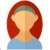Introduction to Algebra
This is a basic subject on matrix theory and linear algebra. Emphasis is given to topics that will be useful in other disciplines, including systems of equations, vector spaces, determinants, eigenvalElectronics and DIY hacker, love to build nifty little toys for fun and profit!
Introduction
This is a basic subject on matrix theory and linear algebra. Emphasis is given to topics that will be useful in other disciplines, including systems of equations, vector spaces, determinants, eigenvalues, similarity, and positive definite matrices.
Share
Modules
Introduction to equations, matrices, subscapes and graphs
Deeper delve into matrices, subspaces, eigen values / vectors and introduction to differential equations
Advanced matrices, matrix operations, compression & pseudo inverse